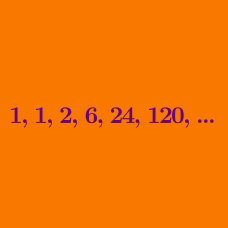Number Theory

# Number Theory Step Up to Level 4 - Set 1

This series of questions helps you understand how to solve the following Level 4 problem about a Diophantine equation:

How many ordered quadruples of positive integers $(w, x, y, z)$ are there such that $w! = x! + y! + z! \quad?$

Step 1: Understanding the problem - Define the terms

Which of the following is an ordered quadruple of positive integers that satisfies the condition

$w! = x! + y! + z! \quad ?$

Step 2: Gathering information - Try small cases

A good way to start is to test small cases to see if we can deduce more information, or even find a solution!

Are there any positive integer solutions to the equation

$w! = x! + y! + z!$

in the case where $w = 1?$

Step 3: Gathering information - Try small cases

In the previous step, we checked the case where $w = 1.$ What happens when we work with variables on the right-hand-side? Since the variables are symmetric, it doesn't matter which one we test.

Are there any positive integer solutions when $z = 2$ to the equation

$w! = x! + y! + z! \quad ?$

Step 4: Review - Explain an observation

Let's review and vocalize what we have learned in steps 2 and 3.

Which variable, when fixed in value, allows us to check finitely many cases to determine if there is a solution to

$w ! = x! + y ! + z! \quad ?$

Step 5: Develop a plan - Explore the idea

From step 4, we want to consider what happens when we restrict the value of $w$. We've already considered the case when $w = 1$ is small. Let's consider what happens for larger values of $w$.

By numerically testing the values, how many positive integer solutions are there to $w! = x! + y! + z!$ with $w = 4$?

Step 6: Review - Explain an observation

Which of the following is the best explanation for why there are no solutions for $w = 4$ to the equation

$w! = x! + y! + z! \quad ?$

Step 7: Put it together - Integrate the information

Given $w \geq 4$, how many positive integer solutions are there to

$w! = x! + y! + z!$

Step 8: Put it together - Answer the problem

How many ordered quadruples of positive integers $(w, x, y, z)$ are there such that $w! = x! + y! + z!\quad ?$

×